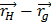# In the cube of side ‘a’ shown in the figure, the vector from the central point of the face ABOD to the central point of the face BEFO will be:(a)a() (b)a() (c)a() (d)a()

## Question ID - 50247 :- In the cube of side ‘a’ shown in the figure, the vector from the central point of the face ABOD to the central point of the face BEFO will be:(a)a() (b)a() (c)a() (d)a()

3537===Next Question :
 Three carnot engines operate in series between a heat source at the temperatureand a heat sink at temperature(see figure). There are two other reservoirs at temperature, as shown with. The three engines are equally efficient if:(a)=;(b)=;(c);(d)=;